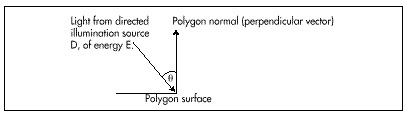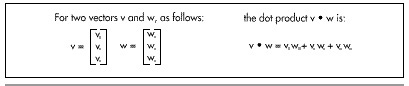So far, the polygons out of which our animated objects have been built have had colors of fixed intensities. For example, a face of a cube might be blue, or green, or white, but whatever color it is, that color never brightens or dims. Fixed colors are easy to implement, but they don’t make for very realistic animation. In the real world, the intensity of the color of a surface varies depending on how brightly it is illuminated. The ability to simulate the illumination of a surface, or shading, is the next feature we’ll add to X-Sharp.

The overall shading of an object is the sum of several types of shading components. Ambient shading is illumination by what you might think of as background light, light that’s coming from all directions; all surfaces are equally illuminated by ambient light, regardless of their orientation. Directed lighting, producing diffuse shading, is illumination from one or more specific light sources. Directed light has a specific direction, and the angle at which it strikes a surface determines how brightly it lights that surface. Specular reflection is the tendency of a surface to reflect light in a mirrorlike fashion. There are other sorts of shading components, including transparency and atmospheric effects, but the ambient and diffuse-shading components are all we’re going to deal with in X-Sharp.

The basic model for both ambient and diffuse shading is a simple one. Each surface has a reflectivity between 0 and 1, where 0 means all light is absorbed and 1 means all light is reflected. A certain amount of light energy strikes each surface. The energy (intensity) of the light is expressed such that if light of intensity 1 strikes a surface with reflectivity 1, then the brightest possible shading is displayed for that surface. Complicating this somewhat is the need to support color; we do this by separating reflectance and shading into three components each—red, green, and blue—and calculating the shading for each color component separately for each surface.

Given an ambient-light red intensity of IAred and a surface red reflectance Rred, the displayed red ambient shading for that surface, as a fraction of the maximum red intensity, is simply min(IAred× Rred, 1). The green and blue color components are handled similarly. That’s really all there is to ambient shading, although of course we must design some way to map displayed color components into the available palette of colors; I’ll do that in the next chapter. Ambient shading isn’t the whole shading picture, though. In fact, scenes tend to look pretty bland without diffuse shading.

Diffuse shading is more complicated than ambient shading, because the effective intensity of directed light falling on a surface depends on the angle at which it strikes the surface. According to Lambert’s law, the light energy from a directed light source striking a surface is proportional to the cosine of the angle at which it strikes the surface, with the angle measured relative to a vector perpendicular to the polygon (a polygon normal), as shown in Figure 54.1. If the red intensity of directed light is IDred, the red reflectance of the surface is Rred, and the angle between the incoming directed light and the surface’s normal is theta, then the displayed red diffuse shading for that surface, as a fraction of the largest possible red intensity, is min (IDred×Rred×cos(θ), 1).

That’s easy enough to calculate—but seemingly slow. Determining the cosine of an angle can be sped up with a table lookup, but there’s also the task of figuring out the angle, and, all in all, it doesn’t seem that diffuse shading is going to be speedy enough for our purposes. Consider this, however: According to the properties of the dot product (denoted by the operator “•”, as shown in Figure 54.2), cos(q)=(v•w)/ |v| × |w| ), where v and w are vectors, q is the angle between v and w, and |v| is the length of v. Suppose, now, that v and w are unit vectors; that is, vectors exactly one unit long. Then the above equation reduces to cos(q)=v•w. In other words, we can calculate the cosine between N, the unit-normal vector (one-unit-long perpendicular vector) of a polygon, and L', the reverse of a unit vector describing the direction of a light source, with just three multiplies and two adds. (I’ll explain why the light-direction vector must be reversed later.) Once we have that, we can easily calculate the red diffuse shading from a directed light source as min(IDred×Rred×(L'• N), 1) and likewise for the green and blue color components.Figure 54.1
Illumination by a directed light sourceFigure 54.2
The dot product of two vectors.

The overall red shading for each polygon can be calculated by summing the ambient-shading red component with the diffuse-shading component from each light source, as in min((IAred×Rred) + (IDred0×Rred×(L0' • N)) + (IDred1×Rred×(L1' • N)) +..., 1) where IDred0 and L0' are the red intensity and the reversed unit-direction vector, respectively, for spotlight 0. Listing 54.2 shows the X-Sharp module DRAWPOBJ.C, which performs ambient and diffuse shading. Toward the end, you will find the code that performs shading exactly as described by the above equation, first calculating the ambient red, green, and blue shadings, then summing that with the diffuse red, green, and blue shadings generated by each directed light source.

Graphics Programming Black Book © 2001 Michael Abrash